Computer Science

# Lab 1a — Introduction to Bit

## Preparation

### 5 minutes

(1) Install Bit. Bit should already be installed on your computer if you successfully executed these commands in the getting started activity:

``conda create -n cs110 matplotlib pytest``

and

``conda run -n cs110 python -m pip install byu_pytest_utils byubit``

If you haven’t been able to set up your environment, see Getting Started

(2) Download the zip file, shown above. Extract its contents and move the `lab1a` folder into your `cs110` folder. Your folder organization should look like this: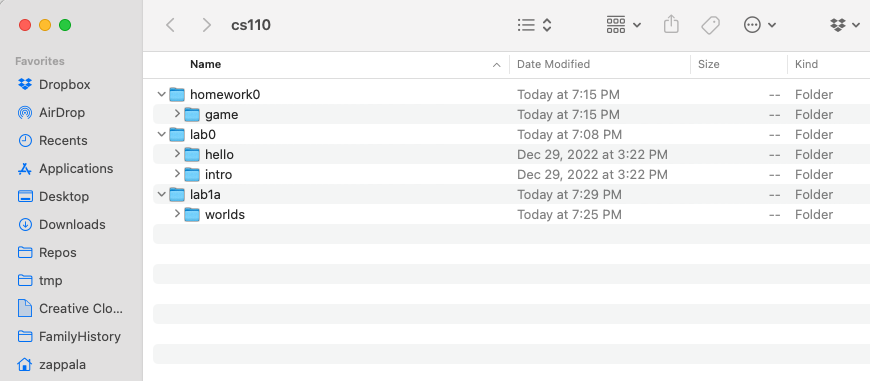Notice that in the `lab1a` folder you have a directory called `worlds`. This directory contains worlds that Bit can operate in. When you see a line like this in a Python file:

``@Bit.worlds('red-dot')``

this tells Bit to use the world called `red-dot.start.txt` inside of the `worlds` folder. It uses `red-dot.finish.txt` to check whether you reached the correct finishing world.

## Exercise 1

### 5 minutes

(a) Can you figure out what this code does?

On a piece of scratch paper, draw out what you think this code will do:

``````from byubit import Bit

@Bit.empty_world(5, 3)
def do_stuff(bit):
bit.paint('blue')
bit.move()
bit.move()
bit.paint('green')
bit.left()
bit.move()
bit.move()
bit.paint('red')

if __name__ == '__main__':
do_stuff(Bit.new_bit)
``````

(b) Run the code

Inside of `lab1a`, create a file called `exercise1.py`. Copy and paste the above code into this file.

Then click on the green triangle next to the main block and run the code: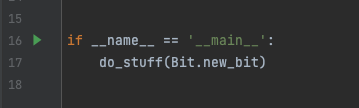See if your drawing was correct!

## Exercise 2

### 5 minutes

(a) Can you figure out what this code does?

On a piece of scratch paper, draw out what you think this code will do:

``````from byubit import Bit

@Bit.empty_world(3, 3)
def do_stuff(bit):
bit.move()
bit.paint('blue')
bit.left()
bit.move()
bit.paint('blue')
bit.move()
bit.left()
bit.move()
bit.paint('blue')
bit.right()
bit.right()
bit.move()
bit.move()
bit.paint('blue')

if __name__ == '__main__':
do_stuff(Bit.new_bit)
``````

(b) Run the code

Inside of `lab1a`, create a file called `exercise2.py`. Copy and paste the above code into this file.

Find the main block and run the code. See if your drawing was correct!

## Exercise 3

### 5 minutes

(a) Can you figure out what this code does?

On a piece of scratch paper, draw out what you think this code will do:

``````from byubit import Bit

@Bit.empty_world(3, 3)
def do_stuff(bit):
bit.paint('blue')
bit.move()
bit.move()
bit.move()
bit.move()
bit.left()
bit.paint('green')
bit.move()
bit.move()
bit.paint('red')

if __name__ == '__main__':
do_stuff(Bit.new_bit)
``````

(b) Run the code

Inside of `lab1a`, create a file called `exercise3.py`. Copy and paste the above code into this file.

Find the main block and run the code. See if your drawing was correct!

## Exercise 4: Red dot

### 10 minutes

You are given some starting code inside of `red_dot.py`:Whenever you see a function that has one statement in it — `pass` — this is a function where you need to write code. The `pass` statement is a special keyword in Python that does literally nothing. It just exists as a placeholder for the code you need to write.

For this problem, Bit starts in an empty 3x3 world: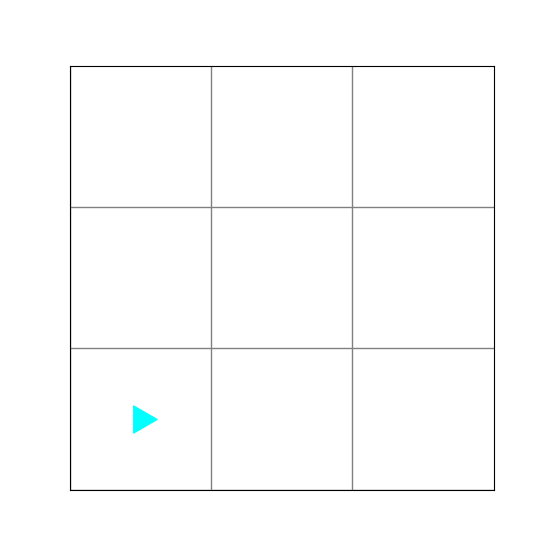Write code in the `red_dot()` function so that the middle square is red. This is what the world should look like when Bit is finished: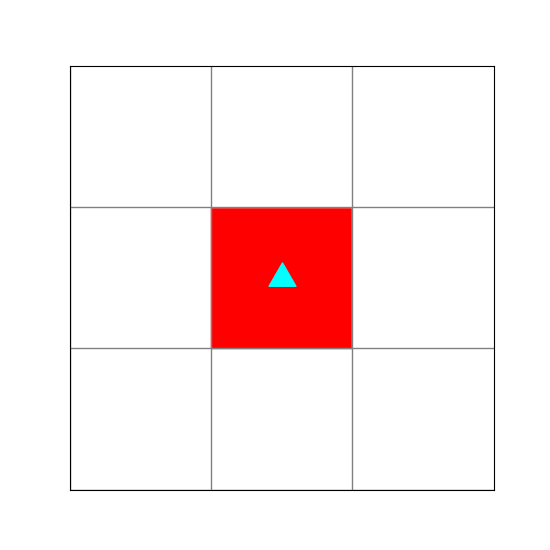1. To get started, delete `pass` and write:
``bit.move()``

Be sure the code is indented because in Python indentation matters:Run this code and you will see that you haven’t solved the problem: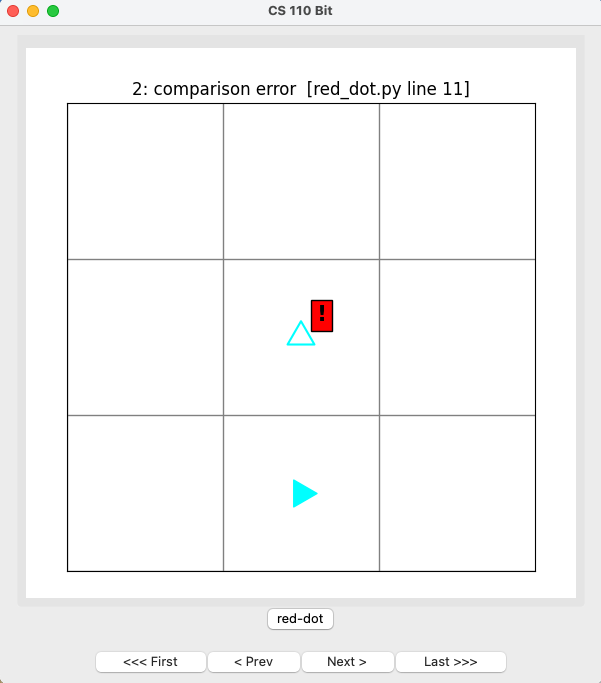There are few important things being shown here:

• The exclamation point on the red background shows that this square should be red.
• The empty Bit in the middle square shows that Bit should be here and pointing up.
• The filled-in Bit in the bottom-middle square shows that Bit is located here after running your code.

Use the buttons at the bottom to see how the code runs:

• `First` will start the beginning
• `Prev` will go to the previous step
• `Next` will go to the next step
• `Last` will go to the end

As you use these buttons, the code being executed at each step is shown at the top of the window.

1. Now write code to solve this problem.

When you get it right, the screen will tell you `compare correct!` at the top:## Exercise 5: All the colors

### 10 minutes

You are given some code in `all-the-colors.py`. Bit starts from a blank 5x5 world:Bit should finish with a world that looks like this: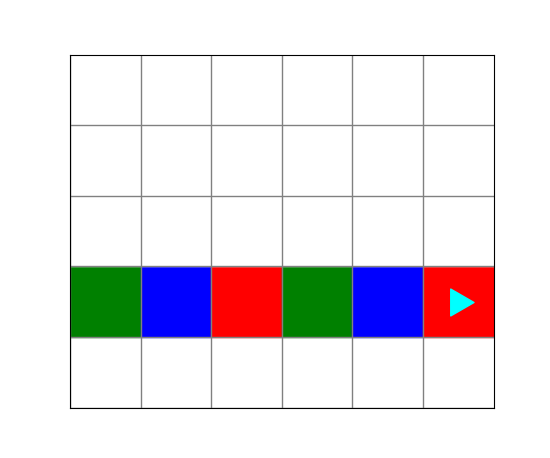Run the code in `all-the-colors.py`. It will finish with a comparison error: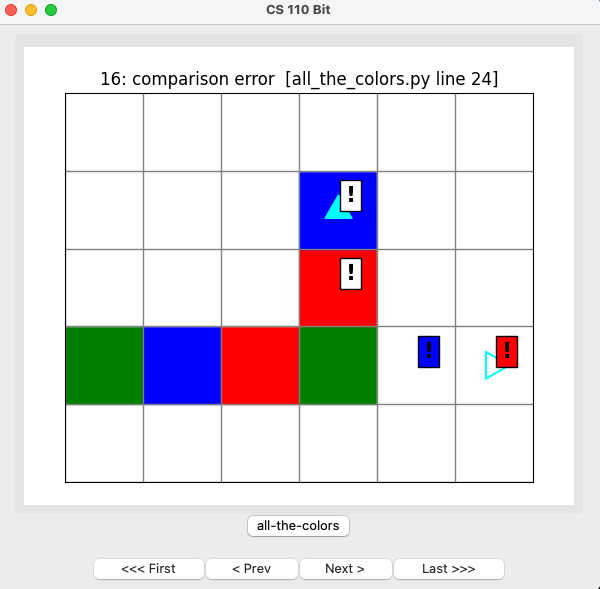• The top two exclamation points have a clear background, showing that these squares should be clear.
• The right two exclamation points have a background that is blue or red, showing that these squares should be blue or red.
• The empty Bit on the right edge shows the location and direction where Bit should be at the end of the problem.

Fix this code so that it works correctly. You should be able to run the code and see that you have it correct: